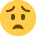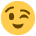# Practice CBT question help

Hi guys,

Having looked at question 1.3 on practice cbt1 for FNPF umpteen times, bashed my head against the wall, looked again, shouted at the cat and finally got a grump on, I've given up. Can anyone please help me out?

We're given

Budgeted O/H £50,000
Budgeted output 5000 units and 50,000 labour hours
Actual output 4000 units and 43,000 labour hours
Actual O/H's £55,000

The fixed overhead efficieny variance is?

My workings from my text book are:
Find the time the actual units produced should have taken (50,000/ 5000 units = 10hrs per unit x 4000 units produced = 40,000)
Minus the actual labour hours to give the labour hour varaince (40,000 - 43,000 = 3,000adverse)
Multiplied by the standard fixed OH absorption rate per hour (3,000 hours x (£50,000 budgeted OH/5000 units = budgeted absorption rate of £10) = £30,000

The answers list the correct figure as £3000I'm sure I'm overly tired and am doing something stupid here - this shouldn't be a difficult question at all but......hmph

Help?

• The first thing to do here is to work out the volume variance as this will equal the capacity and efficiency variance and will also give you a double check in the process.
Once you get to grips with this its not too bad its very mmethodical.
you first went along these lines so i will carry on from there 40,000 compared to 50,000 gives a volume variance of 10,000 and it is adverse because the actual units are less than budgeted,this will equal capacity and efficiency.
Next capacity variance here it is 50,000/50,000=1x43,000=43,000 and compared to budgeted overheads of 50,000 gives a variance of 7,000 adverse because less hours are actually worked.
i hope this helps
basically use units for volume and hours for capacity
• Thanks for your input Omega, but I'm still a bit muddled.

10,000 volume variance minus 7000 labour hours worked variance is indeed 3,000 hours, however the fixed overhead effecieny variance is asked for in £. All the above is a variance in hours?

My text book says to find the efficiency variance in £, I need to multiply the 3,000 labour hour variance by the overhead absorption rate - i.e 10.

The cbt askes for the variance in £ too, yet the answer should apparently be £3,000...?:confused1:
• Everything is a variance from the budgeted overheads which are in £.
So everything is ok.
• forget about overhead absorption rates here,they only matter on labour rate and material price/usage variances.

Hi

im sitting the exam tomorrow and I think my way may help you (hope so)

First I start by asking myself what are we trying to find out? here with this efficiency we are trying to see if the labour force have work more or less efficient than expected?

Then I work out how many hours it should take to make 1 unit here its 50,000 / 5000 i.e. 10 labour hour per unit

Then I multiply the actual units 4000 by this standard 10 = 40,000 hours
Then I write down how many hours it actually took here its 43,000 hours
Work out the difference = 3000 hours (more than expected adverse)

to equate this into pounds we work out the absorption rate here its £50,000 fixed OH divided by 50,000 labour hours = £1.00 per labour hour

The difference 3000 hours multiplied by the Abs rate of £1.00 is £3000

Hope this helps

Wish me luck for the exam in the morning x

Forgot to say "Overheads are divided by the labour hours as we are looking at efficiency"
a) using £50,000 budget and 50,000 hours. Am I confusing hours v overheads?
b) My BPP text book clearly saying I need to multiply the variance in actual hours worked against the overhead absorption rate

You don't mention needing the original overhead absorption rate in your calculations at all.

Thing is the volume variance, 50,000 minus 40,000 isn't in £ is it? My understanding is that the volume variance is 50,000 hours minus the budgeted hours figure adjusted to actual production.
I.e it should have taken 50,000 hours to make 5000 units which is 10 hours per unit. We've only produced 4000 units, so if we'd have still taken 10 hours per unit, production hours would have come in at 40,000. Instead, they've taken 43,000 leaving a variance on 3,000. However at this point, we have't even considered the cost of the overheads - that's all just production hours vs units made.
My text says at this point to multiply the variance in hours by the overhead absorption rate to find the £value of the 3,000 extra hours used.
The logic from my book makes perfect sense to me, but the answer doesn't stack up.
In your answer above, where are you converting the labour hours into £ ?
Omega, you're doing it wrong bud; we did need the o/h absorption rate.

I've just realised I calculated the absorption rate as £50,000 oh divided by 5000 units, rather than £50,000 OH divided by 50,000 labour hours. Doh! Not sure why I was calculating the absorption rate as OH v units.?

Therfore 3000 labour hour variance x£1 OH per hour is £3,000

I wish you all the luck Wad, but it looks like you won't need it!

Thank you:thumbup::thumbup:
• Budgeted overheads are £50,000 everything flows from here.
also,i think my way is best,because you have an automatic double check ie volume variance will equal cap+eff
So,if you know how to work the volume and capacity out you can work the efficency out and have a double check knowing you have arrived at the right answer.
I have just passed my exam exceeding in 7/10 and met in other 3 so i feel i am competent at suggesting these for you.
I have also just looked at my osborne workbooks and my way of working arrives at the right answers.
In the exam for instance you could get one adverse and one favourable and you have to work it out and convince yourself you have the right answer.
• Perhaps I'm being dense, but I don't see how your method can value a variance in hours without considering how much each hour costs i.e the absorption rate.
Anyway, no matter - thanks for your input everyone -I'll go apologise to the catThe difference between the standard hours for the actual production and the actual hours, valued at the standard labour hour absorption rate

Standard hours = 10 x 4000 = 40,000 hours, actual hours 43,000 difference = 3000 valued at standard labour absorption rate = £1.00 total variance £3000 Adverse

Abs rate calculated at £50,000 / 50,000 hours = £1.00

Hope this is better explanation x
• Best to find a way thats suits but some of my fellow study buddies found that using the double check with calculation all sub variances a bit lengthy meaning they ran out of time for the written sections. Good luck everyone am starting to feel anxious but going top try and keep it together as its my last exam then im finished x

The difference between the standard hours for the actual production and the actual hours, valued at the standard labour hour absorption rate

Standard hours = 10 x 4000 = 40,000 hours, actual hours 43,000 difference = 3000 valued at standard labour absorption rate = £1.00 total variance £3000 Adverse

Abs rate calculated at £50,000 / 50,000 hours = £1.00

Hope this is better explanation x
• Thanks fella - I was so almost there originally, I was just calculating the absorption rate on units rather than labour hours.
Hi you made me chuckle when I read fellax think I need to change my user name to something a little more girly x